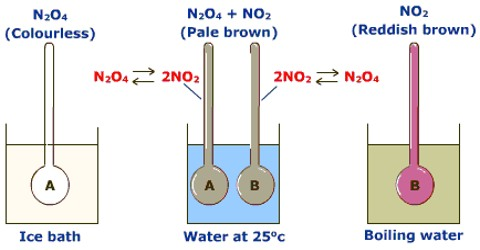Sign-up for a FREE 30-minute Intro to Teaching with 3D and VR here

RegisterCrystal

types of chemical reactions

types of equilibrium

Le- Chateliers principle

# CBSE Class 11 Chemistry Chapter 7 Revision Notes

Chemical equilibrium is the condition in which the concentrations of reactants and products do not vary any more in a chemical process. The rate of forward reaction equals the rate of backward reaction at equilibrium.

# Chapter 7: Equilibrium Revision Notes

Physical and Chemical Processes

• Physical processes cause these changes, but they only impact the physical qualities of the substance being changed, not the chemical makeup or properties.
• Changes in chemical composition and characteristics are involved in chemical processes. A chemical reaction happens whenever a chemical change occurs.

## TYPES OF CHEMICAL REACTIONS

1. Combination Reactions

Two or more chemicals combine to produce a single compound in such reactions.

e.g.,\s2Mg + O2 → 2MgO

2. Decomposition Reactions

These are the reactions. When a chemical decomposes, two or more distinct compounds are produced.

e.g., PCI5 ⇔ PCI3 + CI2

Food digestion is a breakdown event as well.

3. Displacement Reactions

One element or group is displaced by another in these reactions. In actuality, these are redox reactions, such as

Zn(s) + H2SO4

4. Double Displacement or Metathesis Reactions

Precipitation reactions, neutralisation reactions, and other reactions in which two compounds react to generate two new compounds with no change in oxidation state.

AgNO3(aq) + NaCI(aq) + AgCl(s) + NaNO3(aq) + NaNO3(aq) + NaNO3(aq) + NaNO3(aq) + NaNO3(aq) + Na (aq)

## EQUILIBRIUM STATE

• When a reversible process or chemical reaction is carried out in a closed container under certain conditions, some visible attributes such as colour intensity, pressure, and density remain constant.
• Equilibrium is the term used to describe such a condition.

There are several types of equilibrium:

Physical Equilibrium

• Physical equilibrium is established in physical processes such as evaporation of water, melting of solids, dissolving of solutes, and so on. Water with ice
• When everything is in balance, The rate at which ice melts equals the rate at which water freezes.

Chemical Equilibrium

• When a reversible reaction occurs in a closed vessel, a stage is reached when the forward reaction speed matches the backward reaction speed.
• It's the same as chemical equilibrium.
• At equilibrium, the forward reaction rate equals the backward reaction rate.

Characteristics of Chemical Equilibrium

1. Equilibrium can be achieved from any direction.

2. Equilibrium is dynamic in nature, i.e., the response does not end at equilibrium.

3. At equilibrium, the concentrations of different species do not vary.

4. The presence of a catalyst has no effect on the equilibrium state. Catalyst aids in the speedy attainment of balance.

5. The process's observable physical features become constant.

Law of Mass Action

• According to Guldberg and Waage, the rate of a chemical reaction is proportional to the product of the interacting compounds' active masses.
• The rate constants kf and kb are used in this equation.
• At equilibrium, the forward reaction rate equals the backward reaction rate.
• The equilibrium constant is known as Kc.

## TYPES OF EQUILIBRIUM

Homogeneous Equilibrium

• The reactants and products are in the same phase or physical suite in homogenous equilibrium (gaseous or liquid).

2SO3 = 2SO2(g) + O2(g) (g)

Heterogeneous Equilibrium

• Reactants and products exist in two or more physical states or phases in heterogeneous equilibrium.

Fe3O4(s) + 4H2O(g) Fe3O4(s) + 4H2O(g) Fe3O4(s) + 4H2O(g) Fe3O4(s) + 4H2O(g) Fe (g)

## LE- CHATELIER’S PRINCIPLE

• The condition of equilibrium is influenced by three key elements.
• Concentration, temperature, and pressure are the three variables.
• If a system in equilibrium is exposed to a change in concentration, pressure, or temperature, Le – Chatelier's principle asserts that it will lose equilibrium. the equilibrium balance shifts

Effect of Change of Concentration

• If the concentration of one of the reactants is raised at equilibrium.
• In the forward movement, the equilibrium will change, and vice versa.

Effect of Change in Pressure

• Pressure has no influence on equilibria with the same moles of reactants and products.
• N2 + O2 = 2NO, for example.
• When the number of moles changes, the equilibrium shifts in the direction of fewer moles when the pressure is increased, and vice versa, for example, N2 + 3H2 2NH3 [High p. high yield of NH3]

Effect of TemperatureSource:

• When a process is exothermic, the forward reaction is favoured by a low temperature. When a process is endothermic, it is said to be endothermic.
• The production of products is aided by high temperatures.

Effect of Addition of Inert Gas

(i) Under continual strain. if an inert gas is used as a filler It will boost the system's volume. As a result, the equilibrium will change in a direction where the number of moles of gases increases.

(ii) Constant-volume addition of inert gas An inert gas is introduced to maintain the volume of the system constant. The substance's relative molar concentration will not change. As a result, the reaction's equilibrium position is unaltered.

Effect of Catalyst

The presence of a catalyst has no effect on the equilibrium position. It just expedites the process of achieving balance.

Le-Chatelier’s Principle Applicable to Physical Equilibrium

(i) Pressure's effect on solubility: The solubility of gas will increase when pressure rises, and vice versa.

(ii) Solubility as a function of temperature: The absorption of heat causes some things to disintegrate. The solubility of such substances increases as the temperature rises and vice versa, as shown in the dissolution of NH4CI, KCI, KNO3, and other compounds. Calcium acetate and calcium hydroxide dissolve exothermically, therefore their solubility decreases as the temperature rises.

(iii) Pressure's effect on the melting point of ice:

Ice is a kind of liquid water.

Because ice has a larger volume than liquid water, greater pressure will cause ice to melt, according to the Le-Chatelier principle.

Source: